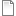# Advanced Scripting: 02 Koch Snowflake

Software:
Tag:
Video Duration:
7 minutes
Author:
Zach Downey

In this tutorial we create a Koch Snowflake.  To do it, we create two methods, CreateTri and CreateKochSnowflake.  This component as a boolean toggle to invert the snowflake.  It works by reversing the polyline direction.  It's kinda cool.  Check out the video and the code.

```//Create an empty list of lines to hold our snowflake curves
List<Line> lines = new List<Line>();
//Call the CreateTri function and pass the radius and flip parameters
//Create an array of Lines by extracting the segements from the triangle
Line[] triangle = tri.GetSegments();
//Add these three lines to the lines List
//Create a temporary list of lines
List<Line> tempList = new List<Line>();
//Begin the while loop
int i = 0;
while(i < num){
//make sure the list is empty by calling the Clear() method
tempList.Clear();
lines.Clear();
i++;
}
A = lines;
}```
```public List<Line> CreateKochSnowflake(List<Line> lines){
List<Line> newLines = new List<Line>();
foreach( Line ln in lines){
//Get the length of the line and 4 points to define new snowflake edge
double tempLength = ln.Length;
Point3d ptStarttemp = ln.PointAt(0.0);
Point3d pt1temp = ln.PointAt(0.333);
Point3d pt2temp = ln.PointAt(0.666);
Point3d ptEndtemp = ln.PointAt(1.0);
//Create a new line and rotate it by 60 degs or PI/3
Line rotatedLine = new Line(pt1temp, pt2temp);
rotatedLine.Transform(Rhino.Geometry.Transform.Rotation(Math.PI / 3, pt1temp));
//Create a new point from the end of the rotatedLine and complete the lines
Point3d triTop = rotatedLine.PointAt(1.0);
Line completeTriLine = new Line(triTop, pt2temp);
Line lnStart = new Line(ptStarttemp, pt1temp);
Line lnEnd = new Line(pt2temp, ptEndtemp);
//Add all of the lines to the newLines list in order
}
return newLines;
}```
```public Polyline CreateTri(double radius, bool reverseTri)
{
List<Point3d> pts = new List<Point3d>();

//Angles for triangle points
const double ang0 = (Math.PI / 2);
const double ang1 = (7 * Math.PI / 6);
const double ang2 = (11 * Math.PI / 6);
//Create the three points of the triangle
//Add the points to the pts list
//Create a new polyline called pl
Polyline pl = new Polyline();
//loop through the list of points(should only be three but will use any number b/c we aren't checking BAD!)
foreach(Point3d pt in pts){
}
//Add a copy of the first point to the end of the list to close the polyline
//Check to see if the polyline should be reversed and if true...call the reverse method
if(reverseTri == true){
pl.Reverse();
}
return pl;
}```
Tutorial Files:kochSnowflake.gh

### Rating

Please rate this tutorial below. Thanks.

## Want to Contribute?

Want to be an author? Drop us a line here we'd love to have you.

Already have a video you'd like to post? Send us a link and we'll get you going.

:)